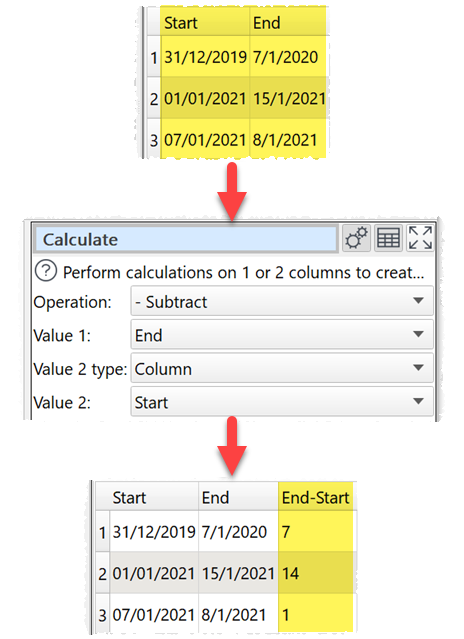﻿ How do I? > Find the difference between dates/datetimes

# Find the difference between dates/datetimes

Navigation:  How do I? >

# Find the difference between dates/datetimes

You can calculate the number off days difference between two dates using the Calculate transform. For example:You can convert a datetime into a date using a transform such as Extract.

Alternatively, you can calculate the difference between two dates or datetimes using Date objects in the Javascript transform.

There are 4 ways to create a Javascript Date object:

Date format

Description

new Date(year, month, day, hours, minutes, seconds, milliseconds)

Specified date and time specified as numeric parameters (January is month 0!).

new Date(text date)

Date and time specified as text.

new Date(milliseconds)

Milliseconds after 1st January 1970.

new Date()

Current date and time.

Notes:

A text date should be in yyyy-mm-dd format.

A Date object always includes a time. If no time is set, then the time is assumed to be midnight GMT.

One and two digit years will be interpreted from 1900.

## Examples

To calculate the number of milliseconds between a date in the 'date' column and 31st Dec 2000:

return new Date( \$(date) ) - new Date( "2000-12-31" );

Or:

return new Date( \$(date) ) - new Date( 2000, 11, 31 );

To calculate the difference between datetimes in the 'start' and 'end' columns in hours:

return ( new Date( \$(end) ) - new Date( \$(start) ) ) / ( 60 * 60 * 1000 );

To calculate how many days ago 'date' occurred (rounded down):

return Math.floor( ( new Date() - new Date( \$(date) ) ) / ( 24 * 60 * 60 * 1000 ) );

For more information see the Javascript documentation.